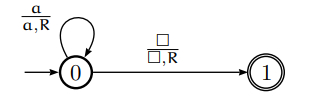# How to use Turing machines to recognize languages in TOC?

A Turing machine (TM) can be formally described as seven tuples −

(Q,X, ∑, δ,q0,B,F)

Where,

• Q is a finite set of states.

• X is the tape alphabet.

• ∑ is the input alphabet.

• δ is a transition function: 𝛿:QxX->QxXx{left shift, right shift}.

• q0 is the initial state.

• B is the blank symbol.

• F is the final state.

A Turing machine T recognises a string x (over ∑) if and only when T starts in the initial position and x is written on the tape,

T halts in a final state.

T is said to recognize a language A, if x is recognised by T and if and only if, x belongs to A.

Be aware that while running a TM, you can also read/write other symbols from/onto a tape, not necessarily only those from the alphabet A.

A Turing machine T does not recognize a string x, if T does not halt in a state that is not final.

There are TMs which for an input don’t halt at all and run forever.

Instantaneous Description

To describe the TM at a given time we need to understand three things, which are given below −

• What is on the tape?

• Where is the tape head?

• What state is the control in?

We will represent this information as follows:

State i − B a a b a b B

Here, the B symbol represents an empty cell (repeated indefinitely to left and right) and the position of the tape head is in red.

Example

For ∑ = {a, b} design a Turing machine that accepts the language denoted by the regular expression a*.

• Step 1 − Starting at the left end of the input, we read each symbol and check that it is a.

• Step 2 − If it is, we continue moving right.

• Step 3 − If we reach a blank symbol without encountering anything but a, we terminate and accept the string.

• Step 4 − If the input contains a b anywhere (the string is not in L(a*)) we halt in a non-final state.

To keep track of the computation, two states 0 (initial) and 1 (final) are sufficient. As the transition function we can use −

T(0, a) = (0, a, R),

T(0,B ) = (1,B , R)

Or we can draw a figure −